# Introduction¶

Snow and Ice cover has been collected in .asc files here by the National Snow and Ice Data Center (NSIDC). One product of interest is the Ice Mapping System (IMS) which takes satellite and field measurements and puts them on a grid. Currently, they have resolutions of about 24x24km 4x4km and 1x1 km. Their website is located here. It has been used to show snow and ice coverage across the northern hemisphere. Rutgers has done a great job displaying this coverage, shown here.

For those like me, who want to display, subsections or time series, or to use this set in their research, accessing and analyzing the data can be cumbersome. First, of all, these files are all zipped and archived on their FTP site. If you want to grab a subset, you have to download them yourself and unzip them individually. If you know how to program, you can just automate this, but unfortunately, the files format changes in the early years, so your automation has to check and see which format the file is using. When you get to the 4x4km resolution, there are some files that contain errors, your automation script may crash if you don't handle these errors accordingly. Finally, when viewing the files, the grid cell areas aren't given. Flatting out the Earth's surface onto a stereographic projection distorts the areas of each grid so that you don't really know what the areas represent. Shown below is one such file, with snow and ice removed.

In :
%matplotlib inline
from IPython.display import Image, display
import os
figures_path = os.path.join(os.sep,'home','tyler','Desktop','itsonlyamodel','content','figures', 'ims')

In :
img_path = os.path.join(figures_path, 'dry_planet_24km.png')
display(Image(filename=img_path))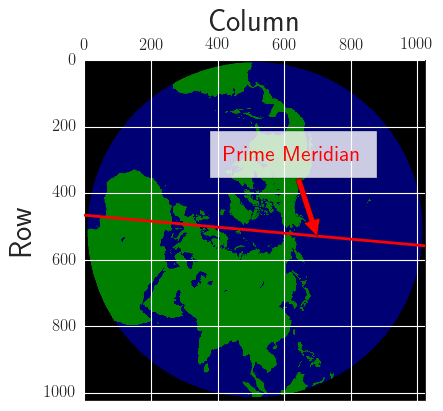Fortunately, I have gone through the pain of these issues and released a project called tibet snow-man on GitHub. I focused on the Tibetan Plateau (TP), but this project can work for any region in the northern hemisphere. The project does the following:

1. Filters for a given lat-long square, for example, the TP region falls within 25-45 latitude and 65-105 longitude

2. Estimates the area of each grid cell using provided lat-long files.

3. Parses out the files locally (use something like FileZilla to download the sets yourself) and flattens them out into a time series. Each year is saved in an HDF5 database that is accessed later on.

4. Sums up snow and ice coverage for a given areas and makes a time series.

5. Generates images showing snow cover on a map projection

The remainder of this article will be used to describe these functionalities. With any luck, this notebook can help those who would like to create videos or time series similar to those shown below. If you have any questions, feel free to email me at tylertucker202@yahoo.com.

In :
img_path = os.path.join(figures_path, 'ts-compare.png')
display(Image(filename=img_path))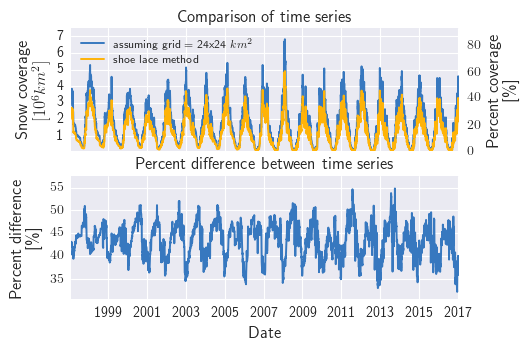First I need to import the following libraries.

In [ ]:
import sys
sys.path.append("../")
from generate_grid_and_area import grid_and_area
import os
import numpy as np
import matplotlib.pyplot as plt
from mpl_toolkits.basemap import Basemap
import pandas as pd
%matplotlib inline
import datetime
import pdb
import seaborn as sns
from matplotlib import rc
from matplotlib import rcParams
import matplotlib.ticker as mtick
rc('text', usetex=True)
rcStyle = {"font.size": 10,
"axes.titlesize": 14,
"axes.labelsize": 14,
'xtick.labelsize': 10,
'ytick.labelsize': 10}
sns.set_context("paper", rc=rcStyle)
sns.set_style("whitegrid", {'axes.grid' : False})
colors = ["windows blue", "amber", "dusty rose", "greyish", "faded green", "dusty purple"]
colorsBW = ["black", "grey"]
sns.set_palette(sns.xkcd_palette(colorsBW))


# 1. Filtering areas¶

The beauty of a data frame is that it can be filtered easily. grid_and_area class contains a data frame object that is filtered in the method reduceLatLong().

First, we instantiate the object grid_maker_filtered. Defines the data frame object and creates a multi index comprised of row and column number.

In :
home_dir = os.getcwd()
data_dir = os.path.join(home_dir,os.pardir,os.pardir,'data')
no_snow_planet_name = 'dry_planet_24km.asc'
lat_grid_filename = 'imslat_24km.bin'
lon_grid_filename = 'imslon_24km.bin'
grid_size = 1024
lat_long_coords_filtered = {'lower_lat': 35,
'upper_lat': 36,
'lower_long': 85,
'upper_long': 86} #set as lower and upper bounds for lat and long
grid_maker_filtered = grid_and_area(lat_long_coords_filtered,data_dir,no_snow_planet_name,grid_size)
lat_long_coords = {'lower_lat': 25,
'upper_lat': 45,
'lower_long': 65,
'upper_long': 105}


Next the lat-long files are added.

In :
grid_maker_filtered.addLatLong(lat_grid_filename, lon_grid_filename)
print('grid maker shape: {}'.format(grid_maker_filtered.df.shape))

grid maker shape: (1048576, 2)

Out:
lat long
row col
0 0 NaN NaN
1 NaN NaN
2 NaN NaN
3 NaN NaN
4 NaN NaN

Note that there are no values for the corner of the map. There is no earth to report, so they are set as NaN. Next step reduces the data frame to the region specified in lat_long_coords_filtered. Additionally, the id column is added, giving each row a unique ID.

In :
grid_maker_filtered.reduceLatLong()
print('grid maker shape: {}'.format(grid_maker_filtered.df.shape))

grid maker shape: (24, 3)

Out:
lat long id
col row
445 760 35.840794 85.096535 0
446 760 35.887020 85.312492 1
447 760 35.932606 85.528885 2
448 760 35.977543 85.745689 3
445 761 35.666016 85.153740 4

I know there is a lot going on here, but for now, just know that the makeSnowHDFStore makes Pandas dataframes that are later used to create time series and plots. It takes a path zipped .asc files as an input and outputs hdf5 files.

# 2. Areas¶

Latitude and Longitude coordinates are given for each point on the grid cell. Currently, the lat-long files for the 1x1km grid have not been released yet.

The class grid_and_area defines creates an instance called grid_maker. Once initialized, addLatLong, reduceLatLong, makeNoSnowMap, and addAreas methods are called to create a data frame object for the Tibetan Plateau.

In :
home_dir = os.getcwd()
data_dir = os.path.join(home_dir, os.pardir, os.pardir, 'data')
no_snow_planet_name = 'dry_planet_24km.asc'
lat_grid_filename = 'imslat_24km.bin'
lon_grid_filename = 'imslon_24km.bin'
lat_long_area_filename = 'lat_long_area.csv'
lat_long_coords = {'lower_lat': 25,
'upper_lat': 45,
'lower_long': 65,
'upper_long': 105} #set as lower and upper bounds for lat and long
grid_size = 1024

grid_maker = grid_and_area(lat_long_coords, data_dir, no_snow_planet_name, grid_size)
grid_maker.reduceLatLong()
grid_maker.makeNoSnowMap()

#tibet falls approximatly in this region.

df_whole = grid_maker.df
df_whole.reset_index(level=df_whole.index.names, inplace=True)

In :
df_whole.shape

Out:
(20607, 13)
In :
df_whole.head(3)

Out:
col row lat long id noSnowMap noSnowMapRBG centroid_lat centroid_long area_points x y area
0 392 683 44.917850 65.165344 0 2 [0, 128, 0] 44.8932 65.3566 {u'top_right': (392, 682), u'bottom_left': (39... 713974.450086 3260701.648276 468.906395
1 391 684 44.647007 65.097160 1 2 [0, 128, 0] 44.6226 65.2872 {u'top_right': (391, 683), u'bottom_left': (39... 701686.887868 3232339.307802 467.064901
2 392 684 44.757946 65.321815 2 2 [0, 128, 0] 44.733 65.5123 {u'top_right': (392, 683), u'bottom_left': (39... 721784.538148 3240670.591263 467.814964

## Check areas with analytical results¶

The areas created are verified to be correct by adding them all up and comparing their collective area with an exact solution.

The Earth is modeled as a sphere of radius $R = 6371 \ km$ The haversine function calculates the great circle distance between two lat-long points in degrees.

$$\Delta \phi = \phi_{2} - \phi_{1} \\ \Delta \lambda = \lambda_2- \lambda_1 \\ a = sin(\frac{\Delta \phi}{2})^{2} + cos(\phi_1) * cos(\phi_2) * sin(\frac{\Delta \lambda}{2})^{2} \\ c = 2 * arcsin(\sqrt(a))$$

Where $\phi$ represents latitude and $\lambda$ represents longitude. and c is the great circle length in degrees.

Next, a semiperimeter area is calculated

$$s = c + \pi + (\phi_{1} + \phi_{2})^{\frac{1}{2}}$$

Finally, The area of a spherical triangle is given by the l'Hullier formula

$$b = \sqrt{ tan(\frac{s}{2}) tan(\frac{s-d}{2}) tan(\frac{s - \pi/2 + \phi_{1}}{2}) tan(\frac{s - \pi/2 + \phi_{2}}{2})} \\ \Delta = 4.0 * arctan(b)$$

where $\Delta$ is the radial area for a spherical triangle.

Treating the longitude points $\lambda_1 = 65^{\circ}$ $\lambda_2 = 105^{\circ}$ and setting $\phi_{1} = \phi_{2}$. Two different spherical triangle areas are obtained for $\phi_top = 45^{\circ}$ $\phi_{bottom} = 25^{\circ}$, $\Delta_{top}$ and $\Delta_{bottom}$ are obtained.

The area of Tibet in $km^2$ is given to be

$$A_{tibet} = R^2(\Delta_{top} - \Delta_{bottom})$$

$A_{tibet}$ is treated as the exact solution and compared to the sum of areas in our data frame

In :
lat_long_area_filename_24 = 'tibet_lat_long_centroids_area_24km.csv'
lat_long_area_filename_4 = 'tibet_lat_long_centroids_area_4km.csv'

from math import radians, cos, sin, asin, sqrt

def haversine_formula(lat1, lat2, lon1, lon2):
"""
Calculate the great circle distance between two points
on the earth (specified in decimal degrees)
"""
# haversine formula
dlon = lon2 - lon1
dlat = lat2 - lat1
a = sin(dlat / 2) ** 2 + cos(lat1) * cos(lat2) * sin(dlon/2) ** 2
c = 2 * asin(sqrt(a))
return c

def semi_perimeter(d, phi_1, phi_2):
return (d  + np.pi  + (phi_1 + phi_2) )* .5

def lhuilier(s, d, phi_1, phi_2):
# uses unit sphere R=1
inner_sq = np.sqrt(np.tan(0.5 * s)
* np.tan(0.5 * (s - d))
* np.tan(0.5 * (s - (np.pi / 2 + phi_1)))
* np.tan(0.5 * (s - (np.pi / 2 + phi_2))))
ans = 4.0 * np.arctan(inner_sq)
return ans

bottom_phi = 25
top_phi = 45
left_lambda = 65
right_lambda = 105

# convert decimal degrees to radians
bottom_phi, top_phi, left_lambda, right_lambda = map(radians, [bottom_phi, top_phi, left_lambda, right_lambda])
R = 6371  # km

d_bottom = haversine_formula(bottom_phi, bottom_phi, left_lambda, right_lambda)
print('bottom length (km): {}'.format(R * d_bottom))
s_bottom = semi_perimeter(d_bottom, bottom_phi, bottom_phi)
E_bottom = lhuilier(s_bottom, d_bottom, bottom_phi, bottom_phi)

d_top = haversine_formula(top_phi, top_phi,left_lambda, right_lambda)
print('top length (km): {} \n'.format(R * d_top))
s_top = semi_perimeter(d_top, top_phi, top_phi)
E_top = lhuilier(s_top, d_top,top_phi, top_phi)

tibet_area = R ** 2 * (E_top - E_bottom)

print('tibet area via 24x24 km grid files: {}'.format(df_24km['area'].sum()))
print('tibet area via Haversine formula: {}'.format(tibet_area))
perc_dif_24 = 100 * (df_24km['area'].sum() - tibet_area) / tibet_area
print('percent difference: {0} \n'.format(perc_dif_24))

print('tibet area via 4x4 km grid files: {}'.format(df_4km['area'].sum()))
print('tibet area via Haversine formula: {}'.format(tibet_area))
perc_dif_4 = 100 * (df_4km['area'].sum() - tibet_area) / tibet_area
print('percent difference: {0}'.format(perc_dif_4))

bottom length (km): 4015.86152343
top length (km): 3112.44504008

tibet area via 24x24 km grid files: 8062815.0957
tibet area via Haversine formula: 8059061.81949
percent difference: 0.0465721232846

tibet area via 4x4 km grid files: 8061716.73857
tibet area via Haversine formula: 8059061.81949
percent difference: 0.0329432773108


Not too shabby overall. Before we move on, I'd like to how IMS stores daily snow data. The .asc files are given in a stereographic projection. The north pole is located at the middle row and column. equator and prime meridian intercection occurs at about the far left column and the middle row. Each point has a designated marker [0,1,2,3,4] O is space, 1 is sea, 2 is land, 3 is ice, and 4 is snow. In the image below, snow and ice was removed, showing space, water and land.

In :
backdrop0 = grid_maker.rbg_no_snow_matrix
fig_size = (6,6)
plt.rcParams["figure.figsize"] = fig_size
fig0 = plt.figure(0)
ax0 = plt.axes()

ax0.set_xlabel('Column')
ax0.set_ylabel('Row')
plt.setp(ax0.get_yticklabels(), fontsize=12)
ax0.xaxis.tick_top()
ax0.xaxis.set_label_position('top')
ax0.imshow(backdrop0)
ax0.set_xlim([0, 1024])
ax0.set_ylim([1024, 0])

tilt = 46
ax0.plot([0, 1024], [512-tilt, 512+tilt], linewidth=2, c='r')
ax0.annotate('Prime Meridian', color = 'r',fontsize=16, xy=(700, 532), xytext=(420, 300),
arrowprops=dict(facecolor='red', shrink=0.01),
)
plt.show()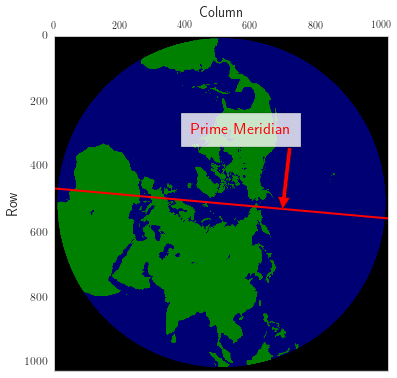In :
#zoom-in-area
zoom = (600,900,200,600)
backdrop = grid_maker.rbg_no_snow_matrix[zoom:zoom,zoom:zoom,:]
#plot of points of interest

llm_x=df_whole['col'].values-zoom
llm_y=df_whole['row'].values-zoom

fig_size = (6,6)
plt.rcParams["figure.figsize"] = fig_size

fig1 = plt.figure(1)
ax1 = plt.axes()
plt.setp(ax1.get_xticklabels(), rotation='vertical')
ax1.imshow(backdrop)
ax1.set_title('Tibetan Plateau region \n embedded in projection grid')
ax1.set_xlabel('Column')
ax1.set_ylabel('Row')
ax1.set_xticklabels([200,250,300,350,400,450,500,550,600])
ax1.set_yticklabels([600,650,700,750,800,850,900])
#rcParams.update({'font.size': 52})
ax1.scatter(llm_x,llm_y, c='w', s=10, marker = 'o',facecolor='0.5', lw = 0)
,
ax1.annotate('Lon = 65$^{\circ}$', color = 'r', fontsize=10, xy=(150, 150), xytext=(140, 125),
rotation=55)
ax1.annotate('Lon = 105$^{\circ}$', color = 'r', fontsize=10, xy=(150, 150), xytext=(315, 145),
rotation=95)

ax1.annotate('Lat = 25$^{\circ}$', color = 'r', fontsize=10, xy=(150, 150), xytext=(200, 210),
rotation=345)
ax1.annotate('Lat = 45$^{\circ}$', color = 'r', fontsize=10, xy=(150, 150), xytext=(220, 115),
rotation=343)
ax1.axis([0,400,300,0])
#ax1.grid(True)
plt.show()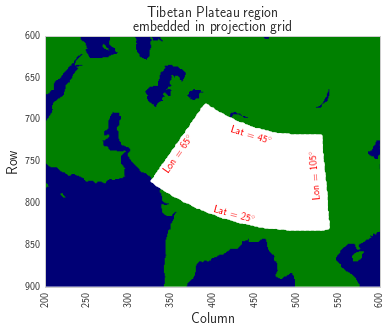## Plot region¶

The Tibetan region is shown in white below. Rows and columns represent the data file's indices.

## Show basemap projection¶

Basemap is relied upon to convert latitude-longitude coordinates from degrees to meters, referenced by some arbitrary point. See Basemap's Website. The code below creates a Lambert Azimuthal Equal Area projection object m, it also sets a blue marble background. The Tibetian region is shown in blue.

In :
fig_size = (6,6)
plt.rcParams["figure.figsize"] = fig_size

def plot_points_on_basemap(filename, df, lat_long_coords, show = True, save = True, width=4500000, height=4000000):
#make map
fig = plt.figure(0)
long_center, lat_center = ((lat_long_coords['upper_long']-lat_long_coords['lower_long'])
/ 2 + lat_long_coords['lower_long'],
(lat_long_coords['upper_lat']-lat_long_coords['lower_lat'])
/ 2 + lat_long_coords['lower_lat'])
m = Basemap(projection='laea',
width = width,
height = height,
resolution='c',lat_0=lat_center,lon_0=long_center)
# this function converts degrees to meters on this reference map
x, y = m(df['long'].values.tolist(), df['lat'].values.tolist())
m.scatter(x, y, marker='.',color='cyan', alpha=.1)
m.bluemarble()
return m

fig2 = plt.figure(2)
filename = 'Tibet-24km'
m = plot_points_on_basemap(filename,df_24km,
lat_long_coords,
show = True,
save = False,
width = 4500000,
height = 4000000)
plt.show()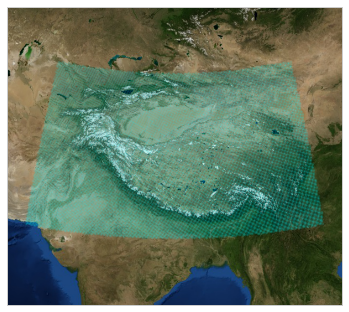## Interactive Tibet Areas¶

Areas over the TP vary substantially. As it turns out, there is quite a substantial difference in areas when traveling along latitude.

Plotly is used to render interactive plots. To export and share, you have to pay an annual subscription, these images may not show up on this blog but You can install plotly on your own environment and run this yourself. Sorry folks. There is a static image below to show the areas on a color scale.

In :
img_path = os.path.join(figures_path, 'areas_of_tibet_grid_i.png')
display(Image(filename=img_path))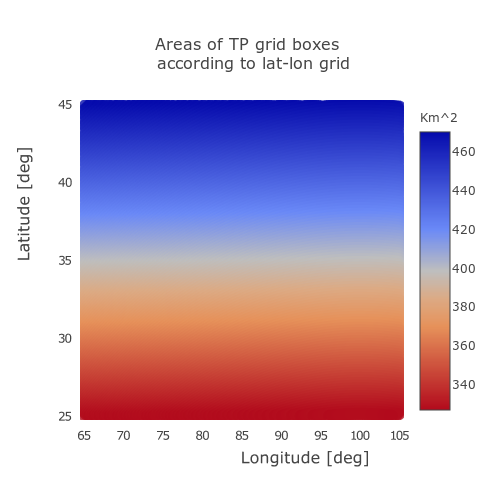## Get max-min ratio¶

The range varies quite a lot and is a source of error. Moral of the story: Each grid area must be calculated separately. Don't assume that each grid is $24x24km^2$ or $4x4 km^2$. In our region, the ratio between the largest and smallest region is about 1.44.

In :
df_whole['area'].max()/df_whole['area'].min()

Out:
1.4398482062172655
In :
from plotly.offline import download_plotlyjs, init_notebook_mode, iplot
import plotly.graph_objs as go
init_notebook_mode()

def make_whole_area_text(X):
return 'Area: %s km^2\
row: %s\
column: %s\
lat: %s\
long: %s\
id: %s'\
% (round(X['area'],2), X['row'], X['col'], round(X['lat'], 3), round(X['long'], 3), X['id'])

scl = [[0,"rgb(5, 10, 172)"],
[0.35,"rgb(40, 60, 190)"],
[0.5,"rgb(70, 100, 245)"],
[0.6,"rgb(90, 120, 245)"],
[0.7,"rgb(106, 137, 247)"],
[0,"rgb(220, 220, 220)"]]
trace = go.Scattergl(
x = df_whole['long'],
y = df_whole['lat'],
text = df_whole.apply(lambda x: make_whole_area_text(x), axis=1),
mode = 'markers',
marker = dict(
size = 8,
opacity = 0.8,
reversescale = True,
autocolorscale = False,
symbol = 'dot',
line = dict(
width=0,
color='rgba(102, 102, 102)'),
colorscale = scl,
cmin = df_whole['area'].min(),
color = df_whole['area'],
cmax = df_whole['area'].max(),
colorbar=dict(
title="Km^2")
)
)

data = [trace]
layout = dict(
autosize=False,
width=500,
height=500,
hovermode=True,
title = 'Areas of TP grid boxes \n according to lat-lon grid',
titlefont=dict(
size=16,
),
colorbar = True,
xaxis=dict(
title='Longitude [deg]',
titlefont=dict(
size=16
),
tickfont=dict(
size = 12
),
),
yaxis=dict(
title='Latitude [deg]',
titlefont=dict(
size=16
),
tickfont=dict(
size = 12
),
),
)
fig3 = dict( data=data, layout=layout)
plot_url = iplot(fig3, validate=False)